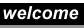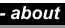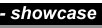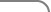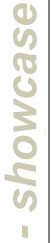Understanding Statistics

We are currently developing a interactive modules to clarify concepts in introductory statistics. We encourage the user to develope a deep understanding with virtual experiments and to apply statistical concepts to current events and everyday issues.

The following modules available for viewing:

 Working with Proportions Learn how proportions behave and how to make statistical decision with proportions.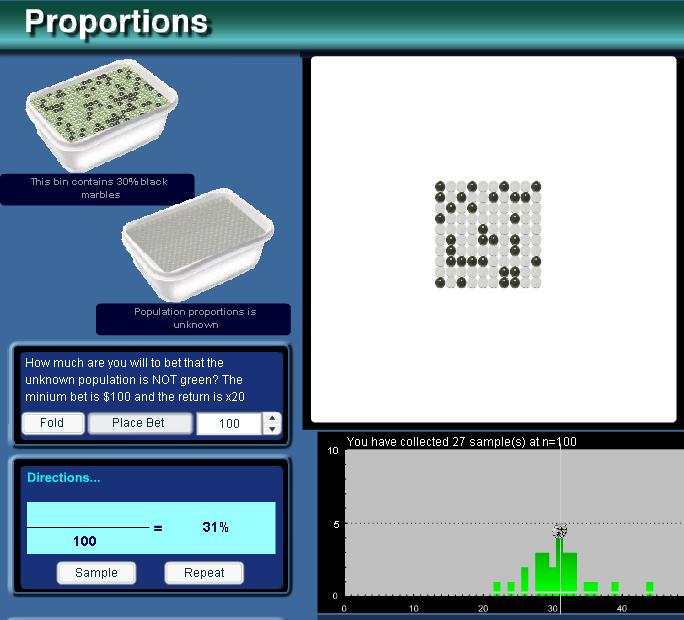Sampling Distribution of Proportions Build your own sampling distribution by scooping marbles from a bin then compare and test your results!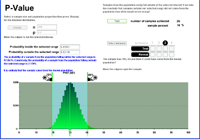Probability and Binomial Distribution Compare the Binomial Distrbution and your sampling distribution of proportions and use on-screen calipers to calculate the probability inside of a selected range.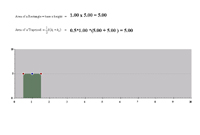Area Under the Bar Change the height and shape of this bar and watch Flash calculate the area under it.Approximating the Binomial Distribution I Adjust the shape of the Binomial Distribution to see if the Normal (Bell-shaped) Curve is a good fit.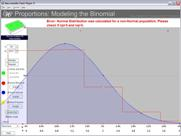Approximating the Binomial Distribution II See when the Normal distribution is a good fit and when the Poisson distribution is a good fit for the Binomial distribution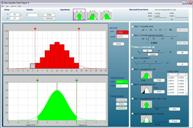z-statistic see how the binomal can be translated to the normal distribution and calculate z-statistics. Working with Means See how populations and sample means behave and learn how to make inferences.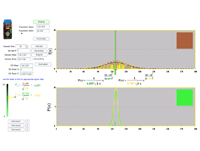Sampling Distribution of Means Take scoops of coffee out of the bag to build your own sampling distribution of means and test a unknown scoop against what you build. If it falls out it's unlike to be the same.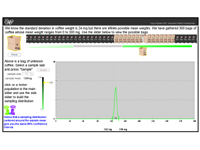Understanding Confidence Intervals If you have a scoop of coffee and it's mean weight, how do you decide whicj bag of coffee it came from? Error and Confidence Intervals: Known Sigma Solidify your understanding of C.I.s by building C.I. and see if and how often it works when it's based on a known population sigma.. Error and Confidence Intervals: Unknown Sigma Solidify your understanding of C.I.s by building C.I. and see if and how often it works when it's based on a sample standard deviation. Making Decisions with z-statitstic Solidify your understanding of C.I.s by building C.I. and see if and how often it works when it's based on a sample standard deviation. Making Decisions with t-statistic Solidify your understanding of C.I.s by building C.I. and see if and how often it works when it's based on a sample standard deviation.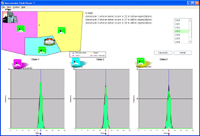Application: student score How do you tell is a school is underforming or if it's variation in the sampling of means? Working with Difference of Proportions Learn how to make decision when you have the difference of two proportions. Sampling Distribution of Difference of Proportions Build your own samplgin distribution by scooping from two bins of marbles and record the difference of proportions. Clinical Data: Vioxx Visualize the Vioxx clinical trial data by putting the points against the expecting sampling distribution of means. Does it fall outside? Working with Probabilities learn the basic operation of working with probability and visualize fundamental theorems Probability Operators Use this tool to visualize and calculate 'and', 'or', 'not', and more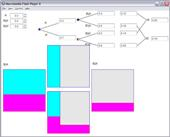Conditional Probability Need to relate one event in terms of another? Bayes Theorem Need to relate one event in terms of another? DeMorgan's Theorem Need to relate one event in terms of another?All images & related site - materials are copyright ©2000 Educated Health Professionals